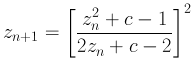## Magnet 1 fractals

These fractals use formulae derived from the study of hierarchical lattices, in the context of magnetic renormalisation transformations. This is useful in an area of theoretical physics that deals with magnetic phase-transitions. In an attempt to clarify the results obtained for real temperatures, the study moved from real to complex numbers, aiming to spot real phase-transitions by finding the intersections of lines representing complex phase-transitions with the real axis. The first people to try this were the two physicists Yang and Lee, who found the situation a bit more complex than first expected, as the phase boundaries for complex temperatures are fractals. The "Magnet 1" fractals, shown on this page, use the equation: